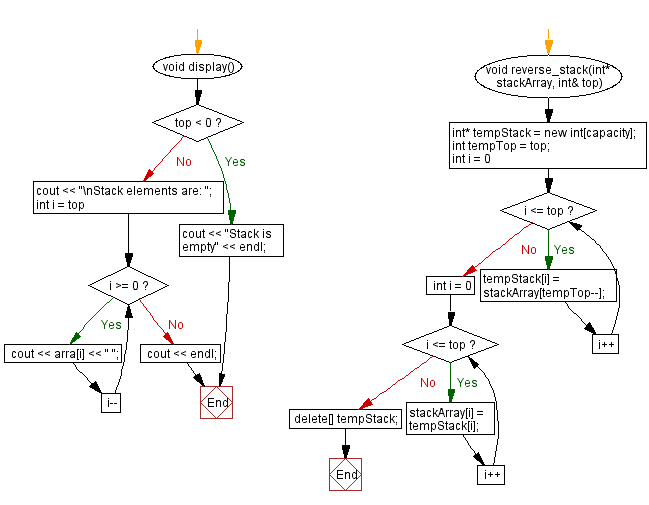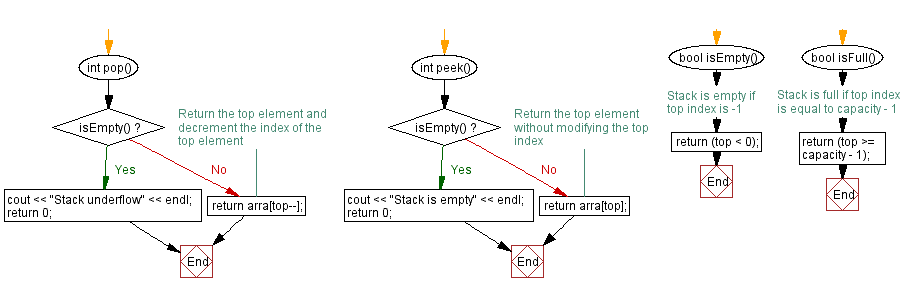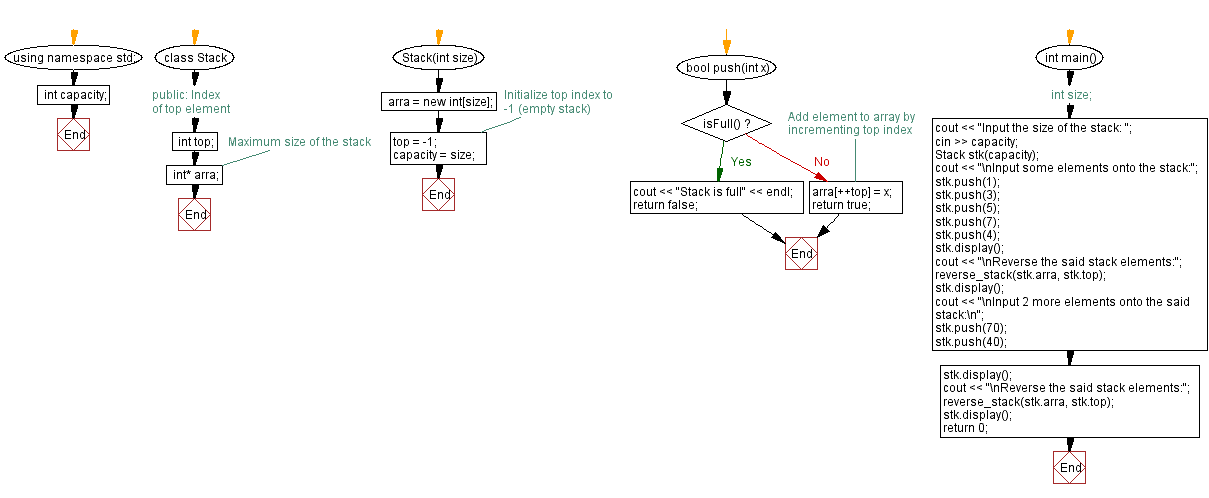﻿ C++ Reverse a stack (using a dynamic array) elements

# C++ Stack Exercises: Reverse a stack (using a dynamic array) elements

## C++ Stack: Exercise-22 with Solution

Write a C++ program that reverses the stack (using a dynamic array) elements.

Test Data:
Input the size of the stack: 8
Input some elements onto the stack:
Stack elements are: 4 7 5 3 1
Reverse the said stack elements:
Stack elements are: 1 3 5 7 4

Sample Solution:

C++ Code:

``````#include <iostream>

using namespace std;
class Stack {
public:
int top;        // Index of top element
int capacity;   // Maximum size of the stack
int* arra;
Stack(int size) {
arra = new int[size];
top = -1;       // Initialize top index to -1 (empty stack)
capacity = size;
}

bool push(int x) {
if (isFull()) {
cout << "Stack is full" << endl;
return false;
}
//Add element to array by incrementing top index
arra[++top] = x;
return true;
}

int pop() {
if (isEmpty()) {
cout << "Stack underflow" << endl;
return 0;
}
//Return the top element and decrement the index of the top element
return arra[top--];
}

int peek() {
if (isEmpty()) {
cout << "Stack is empty" << endl;
return 0;
}
// Return the top element without modifying the top index
return arra[top];
}

bool isEmpty() {
// Stack is empty if top index is -1
return (top < 0);
}

bool isFull() {
// Stack is full if top index is equal to capacity - 1
return (top >= capacity - 1);
}
void display() {
if (top < 0) {
cout << "Stack is empty" << endl;
return;
}
cout << "\nStack elements are: ";
for (int i = top; i >= 0; i--)
cout << arra[i] << " ";
cout << endl;
}
};
void sort_stack(int* stackArray, int& top) {
int temp;
for (int i = 0; i <= top; i++) {
for (int j = i + 1; j <= top; j++) {
if (stackArray[j] > stackArray[i]) {
temp = stackArray[i];
stackArray[i] = stackArray[j];
stackArray[j] = temp;
}
}
}
}
int main() {
int size;
cout << "Input the size of the stack: ";
cin >> size;
Stack stk(size);
cout << "\nInput some elements onto the stack:";
stk.push(1);
stk.push(3);
stk.push(5);
stk.push(7);
stk.push(4);
stk.display();
cout << "\nAfter sorting the said stack elements:";
sort_stack(stk.arra, stk.top);
stk.display();
return 0;
}
``````

Sample Output:

```Input the size of the stack: 8

Input some elements onto the stack:
Stack elements are: 4 7 5 3 1

Reverse the said stack elements:
Stack elements are: 1 3 5 7 4

Input 2 more elements onto the said stack:

Stack elements are: 40 70 1 3 5 7 4

Reverse the said stack elements:
Stack elements are: 4 7 5 3 1 70 40
```

Flowchart:CPP Code Editor: